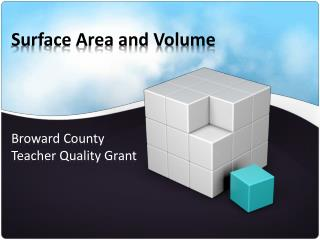DownloadDownload PresentationSurface Area and Volume

# Surface Area and Volume

Télécharger la présentation## Surface Area and Volume

- - - - - - - - - - - - - - - - - - - - - - - - - - - E N D - - - - - - - - - - - - - - - - - - - - - - - - - - -
##### Presentation Transcript

1. Surface Area and Volume Broward County Teacher Quality Grant

2. Big Idea 2: Develop an understanding of and use formulas to determine surface areas and volumes of three-dimensional shapes.

3. Benchmarks MA.7.G.2.1: Justify and apply formulas for surface area and volume of pyramids, prisms, cylinders, and cones. MA.7.G.2.2: Use formulas to find surface areas and volume of three-dimensional composite shapes.

4. Vocabulary • The vocabulary can easily be generated from the reference sheet and the Key. • This will help you not only to review key vocabulary but the symbols for each word.

5. Vocabulary • Take out the vocabulary sheet provided for you and fill in the second column with the definition for each word. • Vocabulary Activity Sheet • Next label the part image in the third column with the letter representing the corresponding vocabulary word. If there is no image draw one.

6. Review Perimeter • Use the worksheets to review circumference and Pi • Rolling a circle • Archemedes estimation of Pi • Use the following PowerPoint to review Perimeter • Perimeter PowerPoint

7. Review Topics GeoGebra activities for Area of Polygons and Circles • Rectangles: • Area of a Rectangle • Parallelograms: • Area of a Parallelogram • Triangles: • Area of a Triangle

8. Review Topics GeoGebra activities for Area of Polygons and Circles • Trapezoids: • Area of a Trapezoid • Circles: • Area of a Circles

9. Review Composite Shapes • PowerPoint for discussing area and perimeter of composite figures. • Composite Shapes PowerPoint

10. Top Back Side 2 Side 1 Front Bottom Rectangular Solid Top Back Side 2 Side 1 Front Height (H) Bottom Breadth (B) Length (L) GeoGebra for a Cube

11. Bases Do the words Bottom and Base mean the same thing?

12. Base of a 3D Figure Bases Triangular Prism Prism: a prism has 2 Bases and the bases, in all but a rectangular prism, are the pair of non-rectangular sides. These sides are congruent, Parallel.

13. Base of a 3D Figure Bases Cylinder GeoGebra Net for Cylinder

14. Base of a 3D Figure Base Pyramid: There is 1 Base and the Base is the surface that is not a triangle.

15. Base of a 3D Figure Base Pyramid: In the case of a triangular pyramid all sides are triangles. So the base is typically the side it is resting on, but any surface could be considered the base.

16. Net Activity Directions sheet Net Sheets Scissors Tape/glue

17. Building Polyhedra

18. GeoGebra Nets Net of a Cube Net of a Square Pyramid Net of a Cylinder Net of a Cone Net of an Octahedron

19. The net ? w ? h h h w h b b ? b b w h h h h w b b w

20. Total surface Area w h w x h w x b b x h b x h b b h h w x h w x b b w + + + + Total surface Area = + = 2(b x h) + 2(w x h) + 2(w x b) = 2(b x h + w x h + w x b)

21. Total surface Area

22. Nets of a Cube GeoGebra Net of a Cube

23. Activity: Nets of a Cube Given graph paper draw all possible nets for a cube. Cube Activity Webpage

24. Nets of a Cube

25. Lateral Area Lateral Area is the surface area excluding the base(s). Net of a Cube

26. Lateral Area Lateral Sides Bases

27. Lateral Area Bases Lateral Surface Net of a cylinder

28. Stations Activity • At each station is the image of a 3D object. Find the following information: • Fill in the boxes with the appropriate labels • Write a formula for your surface area • Write a formula for the area of the base(s) • Write a formula for the lateral area

29. Net handouts and visuals • Printable nets • http://www.senteacher.org/wk/3dshape.php • http://www.korthalsaltes.com/index.html • http://www.aspexsoftware.com/model_maker_nets_of_shapes.htm • http://www.mathsisfun.com/platonic_solids.html • GeoGebra Nets • http://www.geogebra.org/en/wiki/index.php/User:Knote

30. Volume The amount of space occupied by any 3-dimensional object. The number of cubic units needed to fill the space occupied by a solid

31. Volume Activity Grid paper Scissors 1 set of cubes Tape

32. Solid 1

33. Solid 2

34. Solid 3

35. Solid 3

36. Solids 4 & 5 Circular Base Pentagon Base

37. Volume 1cm 1cm 1cm Volume = Base area x height = 1cm2 x 1cm = 1cm3 The number of cubic units needed to fill the space occupied by a solid.

38. Rectangular Prism L • Volume = Base area x height = (b x w) x h = B x h L L • Total surface area = 2(b x w + w x h + bxh)

39. Comparing Volume When comparing the volume of a Prism and a Pyramid we focus on the ones with the same height and congruent bases. h w b h w b

40. Comparing Volume b w h h w w b b